# DTensor Concepts

## Overview

This colab introduces DTensor, an extension to TensorFlow for synchronous distributed computing.

DTensor provides a global programming model that allows developers to compose applications that operate on Tensors globally while managing the distribution across devices internally. DTensor distributes the program and tensors according to the sharding directives through a procedure called Single program, multiple data (SPMD) expansion.

By decoupling the application from sharding directives, DTensor enables running the same application on a single device, multiple devices, or even multiple clients, while preserving its global semantics.

This guide introduces DTensor concepts for distributed computing, and how DTensor integrates with TensorFlow. To see a demo of using DTensor in model training, see Distributed training with DTensor tutorial.

## Setup

DTensor is part of TensorFlow 2.9.0 release, and also included in the TensorFlow nightly builds since 04/09/2022.

````pip install --quiet --upgrade --pre tensorflow`
```

Once installed, import `tensorflow` and `tf.experimental.dtensor`. Then configure TensorFlow to use 6 virtual CPUs.

Even though this example uses vCPUs, DTensor works the same way on CPU, GPU or TPU devices.

``````import tensorflow as tf
from tensorflow.experimental import dtensor

print('TensorFlow version:', tf.__version__)

def configure_virtual_cpus(ncpu):
phy_devices = tf.config.list_physical_devices('CPU')
tf.config.set_logical_device_configuration(phy_devices, [
tf.config.LogicalDeviceConfiguration(),
] * ncpu)

configure_virtual_cpus(6)
DEVICES = [f'CPU:{i}' for i in range(6)]

tf.config.list_logical_devices('CPU')
``````

## DTensor's model of distributed tensors

DTensor introduces two concepts: `dtensor.Mesh` and `dtensor.Layout`. They are abstractions to model the sharding of tensors across topologically related devices.

• `Mesh` defines the device list for computation.
• `Layout` defines how to shard the Tensor dimension on a `Mesh`.

### Mesh

`Mesh` represents a logical Cartisian topology of a set of devices. Each dimension of the Cartisian grid is called a Mesh dimension, and referred to with a name. Names of mesh dimension within the same `Mesh` must be unique.

Names of mesh dimensions are referenced by `Layout` to describe the sharding behavior of a `tf.Tensor` along each of its axes. This is described in more detail later in the section on `Layout`.

`Mesh` can be thought of as a multi-dimensional array of devices.

In a 1 dimensional `Mesh`, all devices form a list in a single mesh dimension. The following example uses `dtensor.create_mesh` to create a mesh from 6 CPU devices along a mesh dimension `'x'` with a size of 6 devices: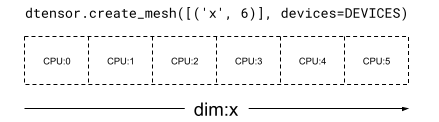``````mesh_1d = dtensor.create_mesh([('x', 6)], devices=DEVICES)
print(mesh_1d)
``````

A `Mesh` can be multi dimensional as well. In the following example, 6 CPU devices form a `3x2` mesh, where the `'x'` mesh dimension has a size of 3 devices, and the `'y'` mesh dimension has a size of 2 devices: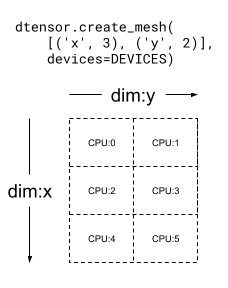``````mesh_2d = dtensor.create_mesh([('x', 3), ('y', 2)], devices=DEVICES)
print(mesh_2d)
``````

### Layout

`Layout` specifies how a tensor is distributed, or sharded, on a `Mesh`.

The rank of `Layout` should be the same as the rank of the `Tensor` where the `Layout` is applied. For each of the `Tensor`'s axes the `Layout` may specify a mesh dimension to shard the tensor across, or specify the axis as "unsharded". The tensor is replicated across any mesh dimensions that it is not sharded across.

The rank of a `Layout` and the number of dimensions of a `Mesh` do not need to match. The `unsharded` axes of a `Layout` do not need to be associated to a mesh dimension, and `unsharded` mesh dimensions do not need to be associated with a `layout` axis.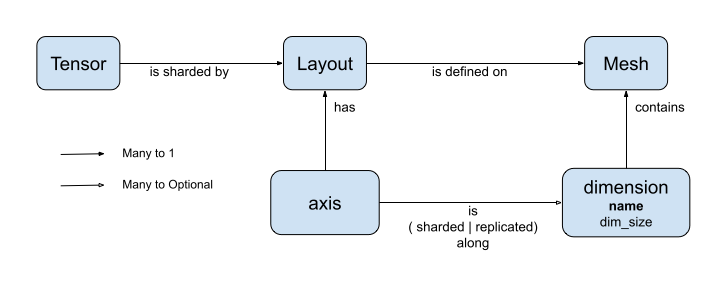Let's analyze a few examples of `Layout` for the `Mesh`'s created in the previous section.

On a 1-dimensional mesh such as `[("x", 6)]` (`mesh_1d` in the previous section), `Layout(["unsharded", "unsharded"], mesh_1d)` is a layout for a rank-2 tensor replicated across 6 devices.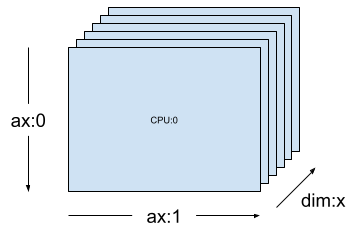``````layout = dtensor.Layout([dtensor.UNSHARDED, dtensor.UNSHARDED], mesh_1d)
``````

Using the same tensor and mesh the layout `Layout(['unsharded', 'x'])` would shard the second axis of the tensor across the 6 devices.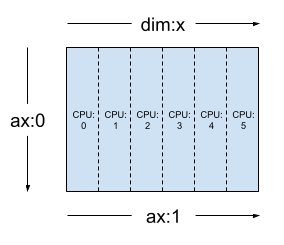``````layout = dtensor.Layout([dtensor.UNSHARDED, 'x'], mesh_1d)
``````

Given a 2-dimensional 3x2 mesh such as `[("x", 3), ("y", 2)]`, (`mesh_2d` from the previous section), `Layout(["y", "x"], mesh_2d)` is a layout for a rank-2 `Tensor` whose first axis is sharded across mesh dimension `"y"`, and whose second axis is sharded across mesh dimension `"x"`.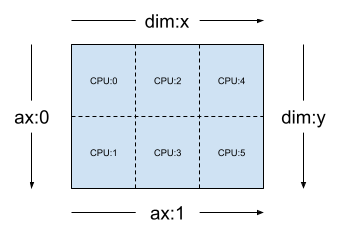``````layout = dtensor.Layout(['y', 'x'], mesh_2d)
``````

For the same `mesh_2d`, the layout `Layout(["x", dtensor.UNSHARDED], mesh_2d)` is a layout for a rank-2 `Tensor` that is replicated across `"y"`, and whose first axis is sharded on mesh dimension `x`.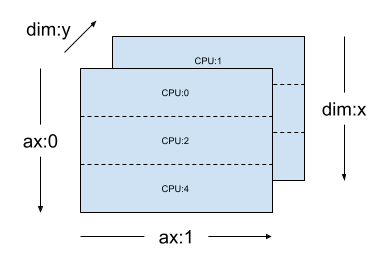``````layout = dtensor.Layout(["x", dtensor.UNSHARDED], mesh_2d)
``````

### Single-Client and Multi-Client Applications

DTensor supports both single-client and multi-client applications. The colab Python kernel is an example of a single client DTensor application, where there is a single Python process.

In a multi-client DTensor application, multiple Python processes collectively perform as a coherent application. The Cartisian grid of a `Mesh` in a multi-client DTensor application can span across devices regardless of whether they are attached locally to the current client or attached remotely to another client. The set of all devices used by a `Mesh` are called the global device list.

The creation of a `Mesh` in a multi-client DTensor application is a collective operation where the global device list is identical for all of the participating clients, and the creation of the `Mesh` serves as a global barrier.

During `Mesh` creation, each client provides its local device list together with the expected global device list. DTensor validates that both lists are consistent. Please refer to the API documentation for `dtensor.create_mesh` and `dtensor.create_distributed_mesh` for more information on multi-client mesh creation and the global device list.

Single-client can be thought of as a special case of multi-client, with 1 client. In a single-client application, the global device list is identical to the local device list.

## DTensor as a sharded tensor

Now let's start coding with `DTensor`. The helper function, `dtensor_from_array`, demonstrates creating DTensors from something that looks like a `tf.Tensor`. The function performs 2 steps:

• Replicates the tensor to every device on the mesh.
• Shards the copy according to the layout requested in its arguments.
``````def dtensor_from_array(arr, layout, shape=None, dtype=None):
"""Convert a DTensor from something that looks like an array or Tensor.

This function is convenient for quick doodling DTensors from a known,
unsharded data object in a single-client environment. This is not the
most efficient way of creating a DTensor, but it will do for this
tutorial.
"""
if shape is not None or dtype is not None:
arr = tf.constant(arr, shape=shape, dtype=dtype)

# replicate the input to the mesh
a = dtensor.copy_to_mesh(arr,
layout=dtensor.Layout.replicated(layout.mesh, rank=layout.rank))
# shard the copy to the desirable layout
return dtensor.relayout(a, layout=layout)
``````

### Anatomy of a DTensor

A DTensor is a `tf.Tensor` object, but augumented with the `Layout` annotation that defines its sharding behavior. A DTensor consists of the following:

• Global tensor meta-data, including the global shape and dtype of the tensor.
• A `Layout`, which defines the `Mesh` the `Tensor` belongs to, and how the `Tensor` is sharded onto the `Mesh`.
• A list of component tensors, one item per local device in the `Mesh`.

With `dtensor_from_array`, you can create your first DTensor, `my_first_dtensor`, and examine its contents.

``````mesh = dtensor.create_mesh([("x", 6)], devices=DEVICES)
layout = dtensor.Layout([dtensor.UNSHARDED], mesh)

my_first_dtensor = dtensor_from_array([0, 1], layout)

# Examine the dtensor content
print(my_first_dtensor)
print("global shape:", my_first_dtensor.shape)
print("dtype:", my_first_dtensor.dtype)
``````

#### Layout and `fetch_layout`

The layout of a DTensor is not a regular attribute of `tf.Tensor`. Instead, DTensor provides a function, `dtensor.fetch_layout` to access the layout of a DTensor.

``````print(dtensor.fetch_layout(my_first_dtensor))
assert layout == dtensor.fetch_layout(my_first_dtensor)
``````

#### Component tensors, `pack` and `unpack`

A DTensor consists of a list of component tensors. The component tensor for a device in the `Mesh` is the `Tensor` object representing the piece of the global DTensor that is stored on this device.

A DTensor can be unpacked into component tensors through `dtensor.unpack`. You can make use of `dtensor.unpack` to inspect the components of the DTensor, and confirm they are on all devices of the `Mesh`.

Note that the positions of component tensors in the global view may overlap each other. For example, in the case of a fully replicated layout, all components are identical replicas of the global tensor.

``````for component_tensor in dtensor.unpack(my_first_dtensor):
print("Device:", component_tensor.device, ",", component_tensor)
``````

As shown, `my_first_dtensor` is a tensor of `[0, 1]` replicated to all 6 devices.

The inverse operation of `dtensor.unpack` is `dtensor.pack`. Component tensors can be packed back into a DTensor.

The components must have the same rank and dtype, which will be the rank and dtype of the returned DTensor. However there is no strict requirement on the device placement of component tensors as inputs of `dtensor.unpack`: the function will automatically copy the component tensors to their respective corresponding devices.

``````packed_dtensor = dtensor.pack(
[[0, 1], [0, 1], [0, 1],
[0, 1], [0, 1], [0, 1]],
layout=layout
)
print(packed_dtensor)
``````

### Sharding a DTensor to a Mesh

So far you've worked with the `my_first_dtensor`, which is a rank-1 DTensor fully replicated across a dim-1 `Mesh`.

Next create and inspect DTensors that are sharded across a dim-2 `Mesh`. The next example does this with a 3x2 `Mesh` on 6 CPU devices, where size of mesh dimension `'x'` is 3 devices, and size of mesh dimension`'y'` is 2 devices.

``````mesh = dtensor.create_mesh([("x", 3), ("y", 2)], devices=DEVICES)
``````

#### Fully sharded rank-2 Tensor on a dim-2 Mesh

Create a 3x2 rank-2 DTensor, sharding its first axis along the `'x'` mesh dimension, and its second axis along the `'y'` mesh dimension.

• Because the tensor shape equals to the mesh dimension along all of the sharded axes, each device receives a single element of the DTensor.
• The rank of the component tensor is always the same as the rank of the global shape. DTensor adopts this convention as a simple way to preserve information for locating the relation between a component tensor and the global DTensor.
``````fully_sharded_dtensor = dtensor_from_array(
tf.reshape(tf.range(6), (3, 2)),
layout=dtensor.Layout(["x", "y"], mesh))

for raw_component in dtensor.unpack(fully_sharded_dtensor):
print("Device:", raw_component.device, ",", raw_component)
``````

#### Fully replicated rank-2 Tensor on a dim-2 Mesh

For comparison, create a 3x2 rank-2 DTensor, fully replicated to the same dim-2 Mesh.

• Because the DTensor is fully replicated, each device receives a full replica of the 3x2 DTensor.
• The rank of the component tensors are the same as the rank of the global shape -- this fact is trivial, because in this case, the shape of the component tensors are the same as the global shape anyway.
``````fully_replicated_dtensor = dtensor_from_array(
tf.reshape(tf.range(6), (3, 2)),
layout=dtensor.Layout([dtensor.UNSHARDED, dtensor.UNSHARDED], mesh))
# Or, layout=tensor.Layout.fully_replicated(mesh, rank=2)

for component_tensor in dtensor.unpack(fully_replicated_dtensor):
print("Device:", component_tensor.device, ",", component_tensor)
``````

#### Hybrid rank-2 Tensor on a dim-2 Mesh

What about somewhere between fully sharded and fully replicated?

DTensor allows a `Layout` to be a hybrid, sharded along some axes, but replicated along others.

For example, you can shard the same 3x2 rank-2 DTensor in the following way:

• 1st axis sharded along the `'x'` mesh dimension.
• 2nd axis replicated along the `'y'` mesh dimension.

To achieve this sharding scheme, you just need to replace the sharding spec of the 2nd axis from `'y'` to `dtensor.UNSHARDED`, to indicate your intention of replicating along the 2nd axis. The layout object will look like `Layout(['x', dtensor.UNSHARDED], mesh)`.

``````hybrid_sharded_dtensor = dtensor_from_array(
tf.reshape(tf.range(6), (3, 2)),
layout=dtensor.Layout(['x', dtensor.UNSHARDED], mesh))

for component_tensor in dtensor.unpack(hybrid_sharded_dtensor):
print("Device:", component_tensor.device, ",", component_tensor)
``````

You can inspect the component tensors of the created DTensor and verify they are indeed sharded according to your scheme. It may be helpful to illustrate the situation with a chart: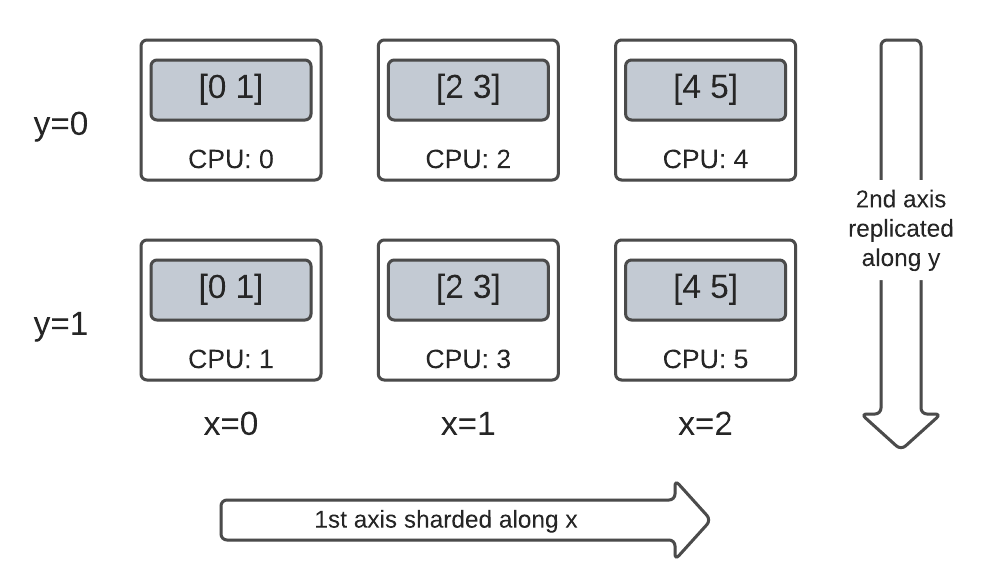#### Tensor.numpy() and sharded DTensor

Be aware that calling the `.numpy()` method on a sharded DTensor raises an error. The rationale for erroring is to protect against unintended gathering of data from multiple computing devices to the host CPU device backing the returned numpy array.

``````print(fully_replicated_dtensor.numpy())

try:
fully_sharded_dtensor.numpy()
except tf.errors.UnimplementedError:
print("got an error as expected for fully_sharded_dtensor")

try:
hybrid_sharded_dtensor.numpy()
except tf.errors.UnimplementedError:
print("got an error as expected for hybrid_sharded_dtensor")
``````

## TensorFlow API on DTensor

DTensor strives to be a drop-in replacement for tensor in your program. The TensorFlow Python API that consume `tf.Tensor`, such as the Ops library functions, `tf.function`, `tf.GradientTape`, also work with DTensor.

To accomplish this, for each TensorFlow Graph, DTensor produces and executes an equivalent SPMD graph in a procedure called SPMD expansion. A few critical steps in DTensor SPMD expansion are:

• Propagating the sharding `Layout` of DTensor in the TensorFlow graph
• Rewriting TensorFlow Ops on the global DTensor with equivalent TensorFlow Ops on the component tensors, inserting collective and communication Ops when necessary
• Lowering backend neutral TensorFlow Ops to backend specific TensorFlow Ops.

The final result is that DTensor is a drop-in replacement for Tensor.

There are 2 ways of triggering DTensor execution:

• DTensor as operands of a Python function, e.g. `tf.matmul(a, b)` will run through DTensor if `a`, `b`, or both are DTensors.
• Requesting the result of a Python function to be a DTensor, e.g. `dtensor.call_with_layout(tf.ones, layout, shape=(3, 2))` will run through DTensor because we requested the output of tf.ones to be sharded according to a `layout`.

### DTensor as Operands

Many TensorFlow API functions take `tf.Tensor` as their operands, and returns `tf.Tensor` as their results. For these functions, you can express intention to run a function through DTensor by passing in DTensor as operands. This section uses `tf.matmul(a, b)` as an example.

#### Fully replicated input and output

In this case, the DTensors are fully replicated. On each of the devices of the `Mesh`,

• the component tensor for operand `a` is `[[1, 2, 3], [4, 5, 6]]` (2x3)
• the component tensor for operand `b` is `[[6, 5], [4, 3], [2, 1]]` (3x2)
• the computation consists of a single `MatMul` of `(2x3, 3x2) -> 2x2`,
• the component tensor for result `c` is `[[20, 14], [56,41]]` (2x2)

Total number of floating point mul operations is `6 device * 4 result * 3 mul = 72`.

``````mesh = dtensor.create_mesh([("x", 6)], devices=DEVICES)
layout = dtensor.Layout([dtensor.UNSHARDED, dtensor.UNSHARDED], mesh)
a = dtensor_from_array([[1, 2, 3], [4, 5, 6]], layout=layout)
b = dtensor_from_array([[6, 5], [4, 3], [2, 1]], layout=layout)

c = tf.matmul(a, b) # runs 6 identical matmuls in parallel on 6 devices

# `c` is a DTensor replicated on all devices (same as `a` and `b`)
print('Sharding spec:', dtensor.fetch_layout(c).sharding_specs)
print("components:")
for component_tensor in dtensor.unpack(c):
print(component_tensor.device, component_tensor.numpy())
``````

#### Sharding operands along the contracted axis

You can reduce the amount of computation per device by sharding the operands `a` and `b`. A popular sharding scheme for `tf.matmul` is to shard the operands along the axis of the contraction, which means sharding `a` along the second axis, and `b` along the first axis.

The global matrix product sharded under this scheme can be performed efficiently, by local matmuls that runs concurrently, followed by a collective reduction to aggregate the local results. This is also the canonical way of implementing a distributed matrix dot product.

Total number of floating point mul operations is `6 devices * 4 result * 1 = 24`, a factor of 3 reduction compared to the fully replicated case (72) above. The factor of 3 is due to the sharding along `x` mesh dimension with a size of `3` devices.

The reduction of the number of operations run sequentially is the main mechansism with which synchronuous model parallelism accelerates training.

``````mesh = dtensor.create_mesh([("x", 3), ("y", 2)], devices=DEVICES)
a_layout = dtensor.Layout([dtensor.UNSHARDED, 'x'], mesh)
a = dtensor_from_array([[1, 2, 3], [4, 5, 6]], layout=a_layout)
b_layout = dtensor.Layout(['x', dtensor.UNSHARDED], mesh)
b = dtensor_from_array([[6, 5], [4, 3], [2, 1]], layout=b_layout)

c = tf.matmul(a, b)
# `c` is a DTensor replicated on all devices (same as `a` and `b`)
print('Sharding spec:', dtensor.fetch_layout(c).sharding_specs)
``````

You can perform additional sharding on the inputs, and they are appropriately carried over to the results. For example, you can apply additional sharding of operand `a` along its first axis to the `'y'` mesh dimension. The additional sharding will be carried over to the first axis of the result `c`.

Total number of floating point mul operations is `6 devices * 2 result * 1 = 12`, an additional factor of 2 reduction compared to the case (24) above. The factor of 2 is due to the sharding along `y` mesh dimension with a size of `2` devices.

``````mesh = dtensor.create_mesh([("x", 3), ("y", 2)], devices=DEVICES)

a_layout = dtensor.Layout(['y', 'x'], mesh)
a = dtensor_from_array([[1, 2, 3], [4, 5, 6]], layout=a_layout)
b_layout = dtensor.Layout(['x', dtensor.UNSHARDED], mesh)
b = dtensor_from_array([[6, 5], [4, 3], [2, 1]], layout=b_layout)

c = tf.matmul(a, b)
# The sharding of `a` on the first axis is carried to `c'
print('Sharding spec:', dtensor.fetch_layout(c).sharding_specs)
print("components:")
for component_tensor in dtensor.unpack(c):
print(component_tensor.device, component_tensor.numpy())
``````

### DTensor as Output

What about Python functions that do not take operands, but returns a Tensor result that can be sharded? Examples of such functions are

For these Python functions, DTensor provides `dtensor.call_with_layout` which eagerly executes a Python function with DTensor, and ensures that the returned Tensor is a DTensor with the requested `Layout`.

``````help(dtensor.call_with_layout)
``````

The eagerly executed Python function usually only contain a single non-trivial TensorFlow Op.

To use a Python function that emits multiple TensorFlow Ops with `dtensor.call_with_layout`, the function should be converted to a `tf.function`. Calling a `tf.function` is a single TensorFlow Op. When the `tf.function` is called, DTensor can perform layout propagation when it analyzes the computing graph of the `tf.function`, before any of the intermediate tensors are materialized.

#### APIs that emit a single TensorFlow Op

If a function emits a single TensorFlow Op, you can directly apply `dtensor.call_with_layout` to the function.

``````help(tf.ones)
``````
``````mesh = dtensor.create_mesh([("x", 3), ("y", 2)], devices=DEVICES)
ones = dtensor.call_with_layout(tf.ones, dtensor.Layout(['x', 'y'], mesh), shape=(6, 4))
print(ones)
``````

#### APIs that emit multiple TensorFlow Ops

If the API emits multiple TensorFlow Ops, convert the function into a single Op through `tf.function`. For example `tf.random.stateleess_normal`

``````help(tf.random.stateless_normal)
``````
``````ones = dtensor.call_with_layout(
tf.function(tf.random.stateless_normal),
dtensor.Layout(['x', 'y'], mesh),
shape=(6, 4),
seed=(1, 1))
print(ones)
``````

Wrapping a Python function that emits a single TensorFlow Op with `tf.function` is allowed. The only caveat is paying the associated cost and complexity of creating a `tf.function` from a Python function.

``````ones = dtensor.call_with_layout(
tf.function(tf.ones),
dtensor.Layout(['x', 'y'], mesh),
shape=(6, 4))
print(ones)
``````

### From `tf.Variable` to `dtensor.DVariable`

In Tensorflow, `tf.Variable` is the holder for a mutable `Tensor` value. With DTensor, the corresponding variable semantics is provided by `dtensor.DVariable`.

The reason a new type `DVariable` was introduced for DTensor variable is because DVariables have an additional requirement that the layout cannot change from its initial value.

``````mesh = dtensor.create_mesh([("x", 6)], devices=DEVICES)
layout = dtensor.Layout([dtensor.UNSHARDED, dtensor.UNSHARDED], mesh)

v = dtensor.DVariable(
initial_value=dtensor.call_with_layout(
tf.function(tf.random.stateless_normal),
layout=layout,
shape=tf.TensorShape([64, 32]),
seed=[1, 1],
dtype=tf.float32))

print(v.handle)
assert layout == dtensor.fetch_layout(v)
``````

Other than the requirement on matching the `layout`, a `DVariable` behaves the same as a `tf.Variable`. For example, you can add a DVariable to a DTensor,

``````a = dtensor.call_with_layout(tf.ones, layout=layout, shape=(64, 32))
b = v + a # add DVariable and DTensor
print(b)
``````

You can also assign a DTensor to a DVariable.

``````v.assign(a) # assign a DTensor to a DVariable
print(a)
``````

Attempting to mutate the layout of a `DVariable`, by assigning a DTensor with an incompatible layout produces an error.

``````# variable's layout is immutable.
another_mesh = dtensor.create_mesh([("x", 3), ("y", 2)], devices=DEVICES)
b = dtensor.call_with_layout(tf.ones,
layout=dtensor.Layout([dtensor.UNSHARDED, dtensor.UNSHARDED], another_mesh),
shape=(64, 32))
try:
v.assign(b)
except:
print("exception raised")
``````

## What's next?

In this colab, you learned about DTensor, an extension to TensorFlow for distributed computing. To try out these concepts in a tutorial, see Distributed training with DTensor.

[{ "type": "thumb-down", "id": "missingTheInformationINeed", "label":"Missing the information I need" },{ "type": "thumb-down", "id": "tooComplicatedTooManySteps", "label":"Too complicated / too many steps" },{ "type": "thumb-down", "id": "outOfDate", "label":"Out of date" },{ "type": "thumb-down", "id": "samplesCodeIssue", "label":"Samples / code issue" },{ "type": "thumb-down", "id": "otherDown", "label":"Other" }]
[{ "type": "thumb-up", "id": "easyToUnderstand", "label":"Easy to understand" },{ "type": "thumb-up", "id": "solvedMyProblem", "label":"Solved my problem" },{ "type": "thumb-up", "id": "otherUp", "label":"Other" }]